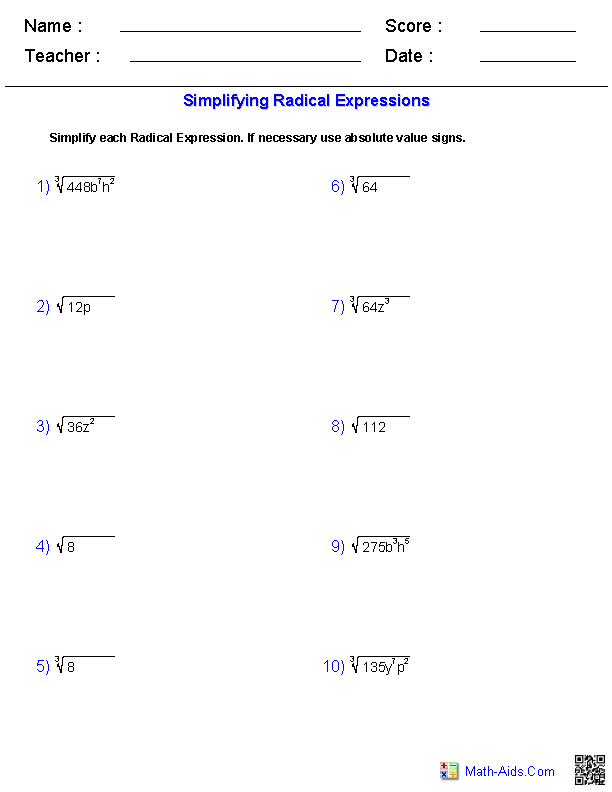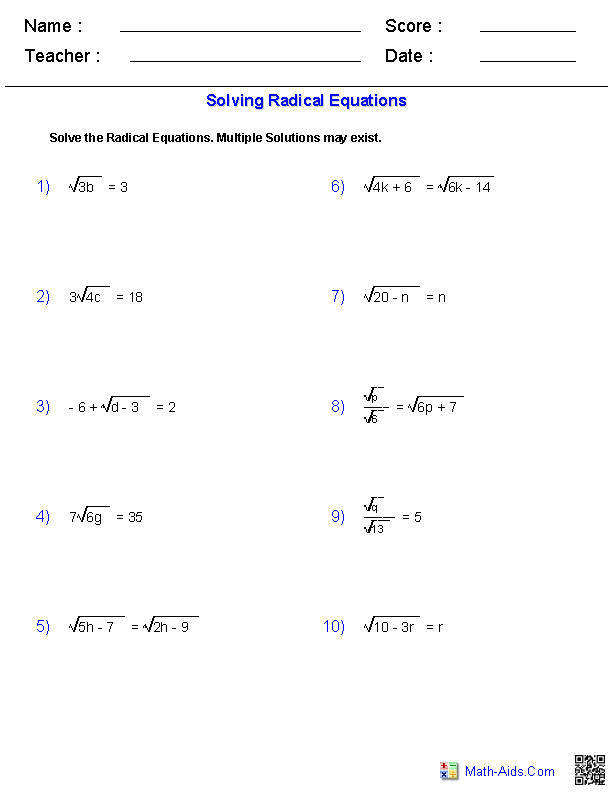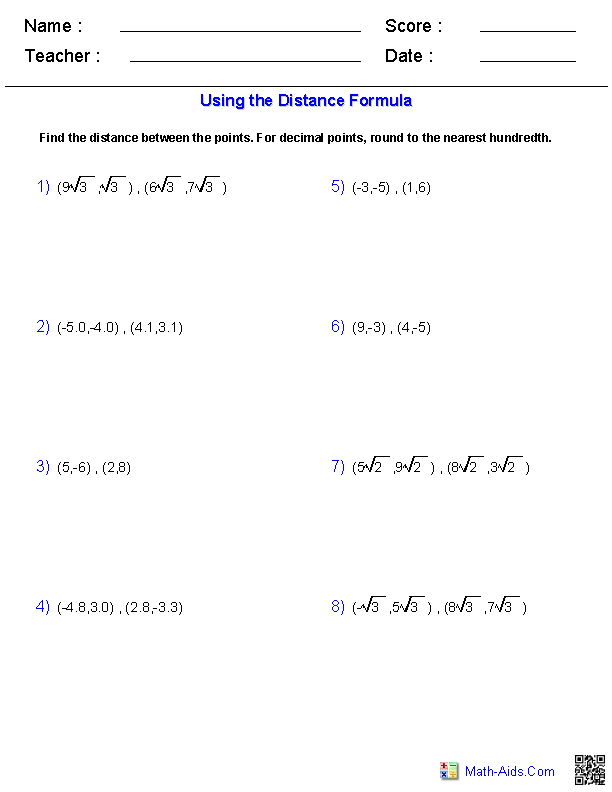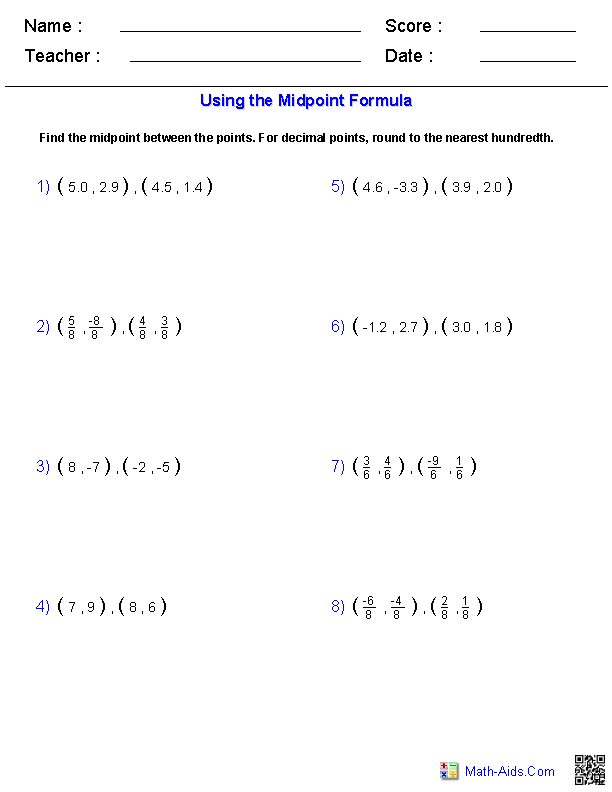#Algebra 1 Worksheets

## Radical Expressions Worksheets

Our Radical Expressions Worksheets are free to download, easy to use, and very flexible.

These Radical Expressions Worksheets are a good resource for students in the 5th Grade through the 8th Grade.

Click here for a Detailed Description of all the Radical Expressions Worksheets.

## Quick Link for All Radical Expressions Worksheets

Click the image to be taken to that Radical Expressions Worksheets.##### MultiplyingRadical Expressions Worksheets##### DividingRadical Expressions Worksheets##### Using the Distance FormulaRadical Expressions Worksheets##### Using the Midpoint FormulaRadical Expressions WorksheetsRecommended Videos

## Detailed Description for All Radical Expressions Worksheets

Simplifying Radical Expressions Worksheets
These Radical Expressions Worksheets will produce problems for simplifying radical expressions. You may select what type of radicals you want to use. These Radical Expressions Worksheets are a good resource for students in the 5th Grade through the 8th Grade.

These Radical Expressions Worksheets will produce problems for adding and subtracting radical expressions. You may select what type of radicals you want to use. These Radical Expressions Worksheets are a good resource for students in the 5th Grade through the 8th Grade.

Multiplying Radical Expressions Worksheets
These Radical Expressions Worksheets will produce problems for multiplying radical expressions. You may select the difficulty for each expression. These Radical Expressions Worksheets are a good resource for students in the 5th Grade through the 8th Grade.

Dividing Radical Expressions Worksheets
These Radical Expressions Worksheets will produce problems for dividing radical expressions. You may select the difficulty for each expression. These Radical Expressions Worksheets are a good resource for students in the 5th Grade through the 8th Grade.

Solving Radical Equations Worksheets
These Radical Expressions Worksheets will produce problems for solving radical equations. You may select the difficulty for each problem. These Radical Expressions Worksheets are a good resource for students in the 5th Grade through the 8th Grade.

Using the Distance Formula Worksheets
These Radical Expressions Worksheets will produce problems for using the distance formula. These Radical Expressions Worksheets are a good resource for students in the 5th Grade through the 8th Grade.

Using the Midpoint Formula Worksheets
These Radical Expressions Worksheets will produce problems for using the midpoint formula. These Radical Expressions Worksheets are a good resource for students in the 5th Grade through the 8th Grade.